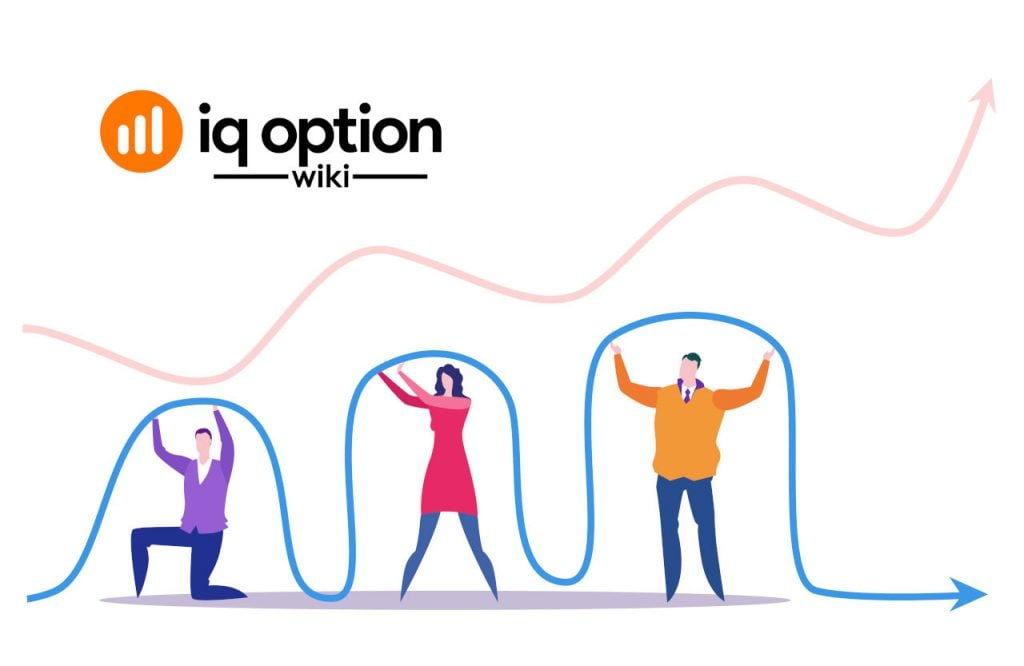Today I will present an indicator that is not so commonly used. It is called the Linear Regression Slope indicator. IQ Option provides many different indicators you can use in your trading venture. We have described many of them so you know how they work and how to make the best of them. But mainly we were focusing on the most famous ones that you can use from the beginning of your trading journey.

## Linear Regression Slope indicator basics

An indicator known as the Linear Regression Slope is an oscillator that measures not only the direction but also the trend's strength. It will help you to forecast the future price movement on the basis of past performance. But first, you have to add the indicator to the chart.

### Adding the Linear Regression Slope indicator to the IQ Option chart

Log in to your IQ Option account and choose the financial instrument you wish to trade with the help of the Linear Regression Slope oscillator. Next, click on the Indicators icon on the left side of the trading platform. You will then see different kinds of indicators. Choose the Trend type and a new list will appear. On this list, you should be able to find the Linear Regression Slope. Click on its name and it will be added to your chart.

The Linear Regression Slope will appear in the separate window below the price bars. You may change its period.

## How to read the Linear Regression Slope indicator?

Our indicator is in a form of a line that oscillates around the 0 line. Generally, there is an uptrend in the market when the Linear Regression Slope readings are positive and it moves over the middle line and the downtrend when the indicator slopes below the line of 0 value.

Rapid movements of the oscillator reveal a trend that is pretty strong.

The higher the period, the more significant measurement. See the picture below. A period of 100 is used to determine long-term trends.

### Trading with the Linear Regression Slope indicator combined with Williams %R

Willimas %R is a momentum oscillator. You can use it next to the Linear Regression Slope in order to identify the best entry points for your trading positions.

The period of the Linear Regression Slope should be significantly longer than Williams %R period. In my example below, I have set, respectively, the periods of 100 and 14.

Williams %R allows catching the oversold and overbought zones. It is enough if it moves below the -80 line or above the -20.

So when you should open a long trade? The best idea is to trade along with the trend so make sure there is an uptrend. Look at the Linear Regression Slope whether its line oscillates above the line of 0 value. If this is the case, check how Williams %R behaves. Enter the trade when it rises from below -80 and crosses this line from below.

You would like to open a short position during the downtrend. This means that the Linear Regression Slope should be fluctuating below the 0 line. Now, let's see where Williams %R lies. If it was above -20 and now it crosses this value on its way down, you ought to enter a short trade.

## Is the Linear Regression Slope indicator good for trading?

The Linear Regression Slope is a lagging indicator that helps to predict the future price movements on the basis of its past performance. It can be successfully used on short and long timeframes.

In order to get a more reliable analysis, you can combine the Linear Regression Slope with some leading indicators as for example Moving Averages or momentum oscillators such as Williams %R.

Remember there is never a guarantee the price will move as expected. Its behaviour is influenced by many things and you have to be always prepared for different scenarios. This is why it is very important you employ good risk management.

Do not forget you can practice new indicators and strategies in a risk-free environment in the IQ Option demo account. Stay there until you get to know the Linear Regression Slope well. The next step will be to earn real money in a live account.

Good luck!

General Risk Warning: The financial products offered by the company carry a high level of risk and can result in the loss of all your funds. You should never invest money that you cannot afford to lose

Click on a star to rate it!

Average rating 3.9 / 5. Vote count: 8

No votes so far! Be the first to rate this post.

As you found this post useful...